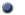Physics-based Sound Synthesis of String Instruments Including Geometric Nonlinearities - Ph.D. thesisThis is homepage of the Ph.D. thesis

Balázs Bank, Physics-based Sound Synthesis of String Instruments Including Geometric Nonlinearities, Ph.D. thesis, Budapest Univeristy of Technology and Economics, Hungary, p. 142, Feb. 2006. URL: http://www.mit.bme.hu/~bank/phd

Materials available in English in pdf format:
the complete Ph.D. thesis

thesis booklet in english
one page abstract

Materials available in Hungarian in pdf format:
tézisfüzet magyarul
egy oldalas kivonat

##Abstract

In this thesis numerous new techniques are developed for the physics-based sound synthesis of string instruments. After reviewing the main physics-based sound synthesis methods, the thesis provides new loss-filter design techniques for the digital waveguide, which is the most common string modeling method. This is followed by proposing efficient modeling techniques for the various parts of the instrument (excitation, strings, body), utilizing the multi-rate approach. Then, the geometric nonlinearities of strings are investigated in detail, with a particular emphasis on the nonlinear generation of longitudinal motion. The obtained theoretical results are applied for the development of string modeling techniques that are able to synthesize the nonlinear effects of the tone.

##Sound Examples for Chapter 5

Original

A recorded C2 piano tone first unaltered, then the transversal components resynthesized by additive synthesis (the longitudinal components and the attack transients are filtered out), and last, the residual containing longitudinal components and attack transients. This example is presented to show the preceptual importance of these components.

Relative Importance of Free and Forced Components (Sec. 5.3.7)

The following examples were generated by the composite string model. Then the longitudinal component was resynthesized by additive synthesis. This made it possible to separate the contribution of the free modes and the phantom partials.

It can be heard that the fast decaying longitudinal modes are masked by the transversal partials after a short time, while the forced response (phantom partials) remains significant. Note that the pitch of the longitudinal component can be heard also in that case when only the phantom partials are present. These examples show that the forced component has a larger perceptual significance and that the pitch preception is mainly determined by the phantom partials and not by the free modes.

##Sound Examples for Chapter 6

The Composite Model (Sec. 6.3.4)

The examples generated by the composite string model based on finite-difference modeling for the transversal vibration and nonlinearly excited resonators for the longitudinal motion:

The sound examples without the longitudinal modeling are presented for easier comparison. These were calculated by using the same parameters for the transversal string model and for the soundboard, but the longitudinal force at the bridge was set to zero. The examples show that the longitudinal component remains an inherent part of the tone, while it is still possible to perceive its pitch, similarly as in the case of real piano tones.

The Resonator-based String Model (Sec. 6.3.5)

This model sounds the same as the composite model of Sec. V B at a lower comutational cost (less than 50%):

Modeling Bidirectional Coupling by Tension Decomposition (Sec. 6.4.2)

The following examples demonstrate the sound of the different regimes of nonlinearity by varying the parameters of a piano string. The example G1 forte tone with realistic parameters is here.

The examples demonstrated by the following wav files are also available as a ppt slide, where the different regimes of nonlinearity are plotted graphically.

Here the amplitude of the string excitation was set to 10 % of its real value. As a result, only the linear transverse components are present.

This is an example with normal amplitude, but due to the large longitudinal/transverse fundamental frequency ratio the longitudinal modes are not excited. Thus, the tension is spatially uniform along the string, and the tension variation leads to double frequency terms in the longitudinal force, but it is to small too act back to the transverse vibration. Note that although the phantom partials can be easily found in the spectrum, for this particular amplitude of vibration they are not audible, as they are masked by the normal partial series.

This is an example with five times higher amplitude. As before, due to the large longitudinal/transverse fundamental frequency ratio the longitudinal modes are not excited. Thus, the tension is spatially uniform along the string, but now the tension variation is large enough to influence the transverse vibration. The audible effect is a pitch glide showing the continous decrease of tension.

This is the G1 forte tone with realistic parameters, meaning that due to the longitudinal/transverse fundamental frequency ratio is in the order of 20, the longitudinal components are excited around and above resonance, leading to a spatially nonuniform tension. However, because of the moderate amplitude of vibration, the tension variation does not influence the transverse vibration significantly. The main perceptual effect is the metallic sound due to the longitudinal components.

The same as above but with five times higher excitation force. Here neither the tension is spatially unifrom along the string, nor the tension variation is negligible in comparison to the tension at rest. As a result, the transverse and longitudinal polarizations interact with each other, leading to the main perceptual effect of the metallic longitudinal components and to a pitch glide.

Other online publications